Example Questions

Example Question #1 : Quadratic Inequalities

Solve the following inequality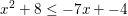No Solution

All Real Numbers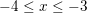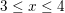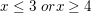Explanation:

We begin by moving all of our terms to the left side of the inequality.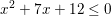We then factor.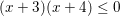That means our left side will equal 0 when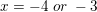.  However, we also want to know the values when the left side is less than zero.  We can do this using test regions.  We begin by drawing a number line with our two numbers labeled.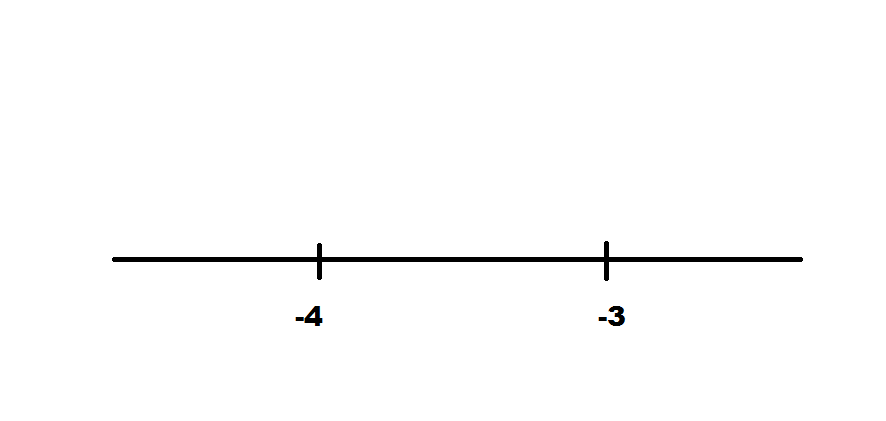We notice that our two numbers divide our line into three regions.  We simply need to try a test value in each region.  We begin with our leftmost region by selecting a number less than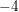.  We then plug that value into the left side of our inequality to see if the result is positive or negative.  Any value (such as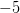) will give us a positive value.

We then repeat this process with the center region by selecting a value between our two numbers.  Any value (such as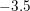) will result in a negative outcome.

Finally we complete the process with the rightmost region by selecting a value larger than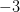.  Any value (such as) will result in a positive value.

We then label our regions accordingly.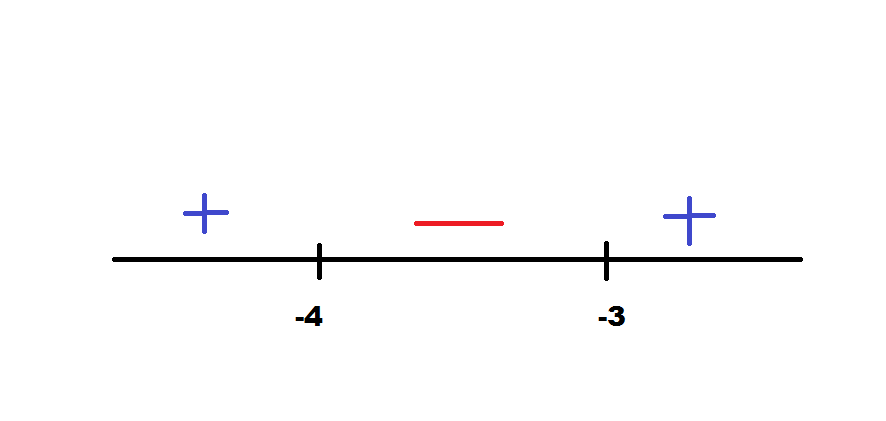Since we want the result to be less than zero, we want the values between our two numbers.  However, since our left side can be less than or equal to zero, we can also include the two numbers themselves.  We can express this asExample Question #1 : Solving Inequalities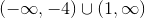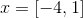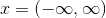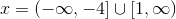Explanation:

We begin by solving the equation for its zeros. This is done by changing the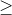sign into ansign.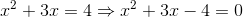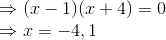Since we know the zeros of the equation, we can then check the areas around the zeros since we naturally have split up the real line into three sections :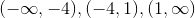First we check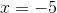Therefore, the first interval can be included in our answer. Additionally, we know that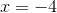satisfies the equation, therefore we can say with certainty that the interval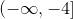is part of the answer.

Next we check something in the second interval. Let, then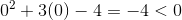Therefore the second interval cannot be included in the answer.

Lastly, we check the third interval. Let, then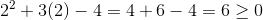Which does satisfy the original equation. Therefore the third interval can also be included in the answer. Since we know thatsatisfies the equation as well, we can include it in the interval as such: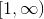Therefore,Example Question #1 : Solving Inequalities

Solve: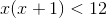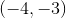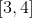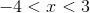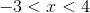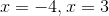Explanation:

Method 1:

1) Multiply-out the left side then rewrite the inequality as an equation: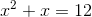2) Now rewrite as a quadratic equation and solve the equation: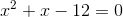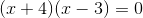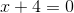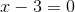3) Next set up intervals using the solutions and test the original inequality to   see where it holds true by using values foron each interval.

4) The interval betweenandholds true for the original inequality.

5) Solution:Method 2:

Using a graphing calculator, find the graph.  The function is below the x-axis (less than) for the x-values.  Using interval notation for,.

Method 3:

For the inequality, the variable expression in terms ofis less than, and an inequality has a range of values that the solution is composed of.  This means that each of the solution values forare strictly between the two solutions of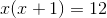.  'Between' is for a 'less than' case, 'Outside of' is for a 'greater than' case.

All Precalculus Resources